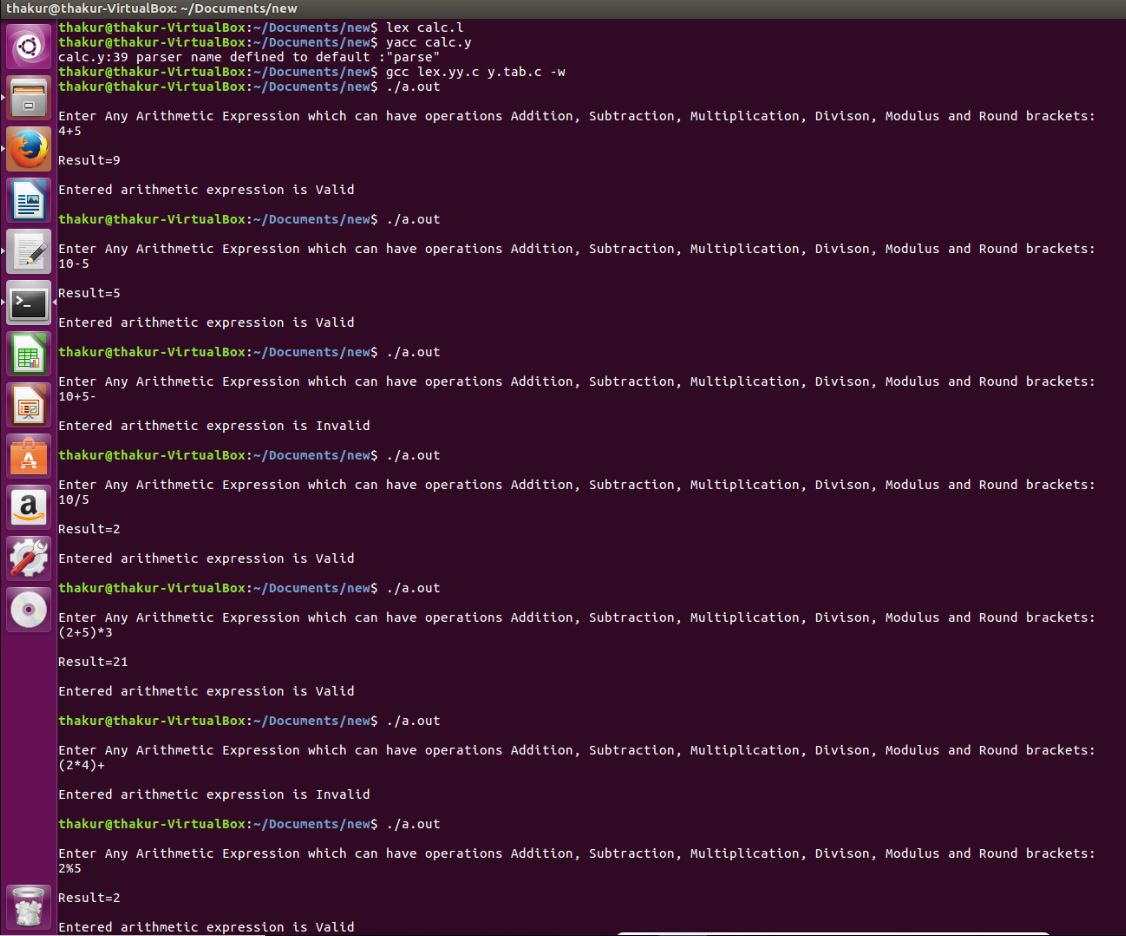Related Articles

# YACC program to implement a Calculator and recognize a valid Arithmetic expression

• Last Updated : 26 Aug, 2020

Problem: YACC program to implement a Calculator and recognize a valid Arithmetic expression.

Explanation:
Yacc (for “yet another compiler compiler.”) is the standard parser generator for the Unix operating system. An open source program, yacc generates code for the parser in the C programming language. The acronym is usually rendered in lowercase but is occasionally seen as YACC or Yacc.

Examples:

```Input: 4+5
Output: Result=9
Entered arithmetic expression is Valid

Input: 10-5
Output: Result=5
Entered arithmetic expression is Valid

Input: 10+5-
Output:
Entered arithmetic expression is Invalid

Input: 10/5
Output: Result=2
Entered arithmetic expression is Valid

Input: (2+5)*3
Output: Result=21
Entered arithmetic expression is Valid

Input: (2*4)+
Output:
Entered arithmetic expression is Invalid

Input: 2%5
Output: Result=2
Entered arithmetic expression is Valid ```

Lexical Analyzer Source Code:

 `%{``   ``/* Definition section */``  ``#include``  ``#include "y.tab.h"``  ``extern` `int` `yylval;``%}`` ` `/* Rule Section */``%%``[0-9]+ {``          ``yylval=``atoi``(yytext);``          ``return` `NUMBER;`` ` `       ``}``[\t] ;`` ` `[\n] ``return` `0;`` ` `. ``return` `yytext;`` ` `%%`` ` `int` `yywrap()``{`` ``return` `1;``}`

Parser Source Code :

 `%{``   ``/* Definition section */``  ``#include``  ``int` `flag=0;``%}`` ` `%token NUMBER`` ` `%left ``'+'` `'-'`` ` `%left ``'*'` `'/'` `'%'`` ` `%left ``'('` `')'`` ` `/* Rule Section */``%%`` ` `ArithmeticExpression: E{`` ` `         ``printf``(``"\nResult=%d\n"``, \$\$);`` ` `         ``return` `0;`` ` `        ``};`` ``E:E``'+'``E {\$\$=\$1+\$3;}`` ` ` ``|E``'-'``E {\$\$=\$1-\$3;}`` ` ` ``|E``'*'``E {\$\$=\$1*\$3;}`` ` ` ``|E``'/'``E {\$\$=\$1/\$3;}`` ` ` ``|E``'%'``E {\$\$=\$1%\$3;}`` ` ` ``|``'('``E``')'` `{\$\$=\$2;}`` ` ` ``| NUMBER {\$\$=\$1;}`` ` ` ``;`` ` `%%`` ` `//driver code``void` `main()``{``   ``printf``("\nEnter Any Arithmetic Expression which ``                   ``can have operations Addition, ``                   ``Subtraction, Multiplication, Division, ``                          ``Modulus and Round brackets:\n");`` ` `   ``yyparse();``   ``if``(flag==0)``   ``printf``(``"\nEntered arithmetic expression is Valid\n\n"``);``}`` ` `void` `yyerror()``{``   ``printf``(``"\nEntered arithmetic expression is Invalid\n\n"``);``   ``flag=1;``}`

Output:Attention reader! Don’t stop learning now. Get hold of all the important CS Theory concepts for SDE interviews with the CS Theory Course at a student-friendly price and become industry ready.

My Personal Notes arrow_drop_up